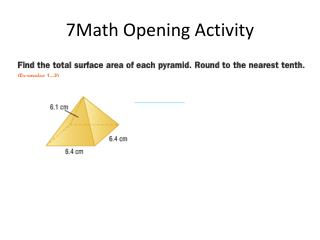# 7Math Opening Activity - PowerPoint PPT PresentationDownload Presentation7Math Opening Activity

7Math Opening Activity
Download Presentation## 7Math Opening Activity

- - - - - - - - - - - - - - - - - - - - - - - - - - - E N D - - - - - - - - - - - - - - - - - - - - - - - - - - -
##### Presentation Transcript

1. 7Math Opening Activity

2. Chapter 8 Lesson 8 Volume and Surface Area of Composite Figures

3. Objective: Students will find the volume and surface area of composite figures.

4. The volume of a composite figure can be found by separating the figure into solids whose volumes you know how to find. Example 1: Find the volume of the composite figure. 10 in Remember, the volume formula for a rectangular prism is: 14 in 7 in 12in 16 in You can separate this figure into two rectangular prisms: 14in 7 in 12 in 12 in 10 in 6 in Add the two volumes together to get the total volume: 1680+504=2184 square inches

5. Example 2: Find the volume of the composite figure: 7.2 ft 6 ft 8 ft 7 ft 8 ft This figure can be separated into a square pyramid and a rectangular prism: 7.2 ft 8 ft 7 ft 8 ft 7 ft 8 ft To get the total volume of the figure, add the two volumes together:

6. You can also find the surface area of composite figures by finding the areas of the faces that make up the composite figure. Example 3:Find the surface area of the following figure: 10 in 14 in 7 in 12 in 16 in We will break this composite shape into its separate faces, find the area of each face, and then add all the areas together to find the total surface area of the composite figure.

7. Example 3 continued- Front Face/Back Face: 10 in This is the shape of the front and back face, so we can find the area of one face and multiply by two because there are two faces. 14 in 7 in 16 in This face can also be separated into two rectangles: To find the total area of the front and back face together, multiply the area of the front face by 2 since the back face has the same dimensions: 10 in 6 in 14 in 7 in A=lw A=lw =(14)(10) =(6)(7) Total area of the front face: 140+ 42=

8. Example 3 continued- Left Face and Right Faces: Left face: Right faces: Both right faces are the same dimensions so we can get the area of one and multiply by 2. 7 in 14 in 7 in 12 in 12 in A= lw A=lw =(14)(12) =(7)(12) Total area of both right faces is:

9. Example 3 continued- top and bottom faces: Bottom face: Top face: 12 in 12in 16 in 10 in A=lw A=lw =(12)(16) =(12)(10)

10. Example 3 continued-total surface area: To find total surface area we need to add all the face areas together. Left/right face Top face 364 + 168 + 192 + 120 = Front and back faces bottom face